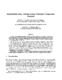## Quantifiable Data Mining Using Principal Component Analysis##### Files
CS-TR-3754.ps(676.72 KB)
CS-TR-3754.pdf(567.98 KB)
Association Rule Mining algorithms operate on a data matrix (e.g., customers x products) to derive rules. We propose a single-pass algorithm for mining linear rules in such a matrix based on Principal Component Analysis. PCA detects correlated columns of the matrix, which correspond to, e.g., products that sell together. The first contribution of this work is that we propose to quantify the goodness'' of a set of discovered rules. We define the guessing error'': the root-mean-square error of the reconstructed values of the cells of the given matrix, when we pretend that they are unknown. The second contribution is a novel method to guess missing/hidden values from the linear rules that our method derives. For example, if somebody bought $10 of milk and$3 of bread, our rules can guess'' the amount spent on, say, butter. Thus, we can perform a variety of important tasks such as forecasting, what-if' scenarios, outlier detection, and visualization. Moreover, we show that we can compute the principal components with a single pass over the dataset. Experiments on real datasets (e.g., NBA statistics) demonstrate that the proposed method consistently achieves a `guessing error'' of up to 5 times lower than the straightforward competitor. (Also cross-referenced as UMIACS-TR-97-13)